# What Factors Cause Strokes?Photo by National Cancer Institute on Unsplash

A stroke occurs when a blood vessel that carries blood or nutrients to the brain is blocked or bursts. There are over 200,000 cases of strokes each year in the United States and according to the World Health Organization, it is the 2nd leading cause of death globally.

In this project, I will be exploring which input parameters correlate with strokes and ultimately build a predictive model to see how likely a patient will get a stroke. The following analysis is done with the dataset found here.

# Exploratory Data Analysis

I first did an exploratory data analysis to understand the dataset I will be working with.

There are 5110 rows of data with the 12 different attributes listed below:

I first graphed a bar chart count plot using all the categorical features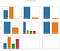Count plot of all categorical features

We can observe that a higher proportion of females are represented and most patients do not have hypertension or heart disease. Most patients have also been married and work in the private sector.

Next, I graphed a distribution plot of the continuous features.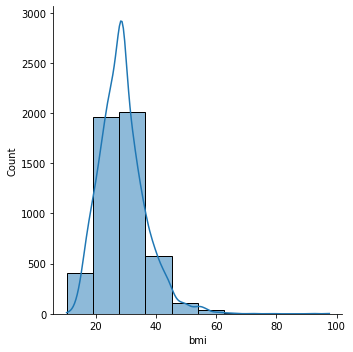Distribution plot of continuous features

We can observe that the majority of the patients are in the 40–60 age range, have an average glucose level of under 100 and a BMI between 20–40.

Then I chose to plot the same graphs, but using only stroke patients.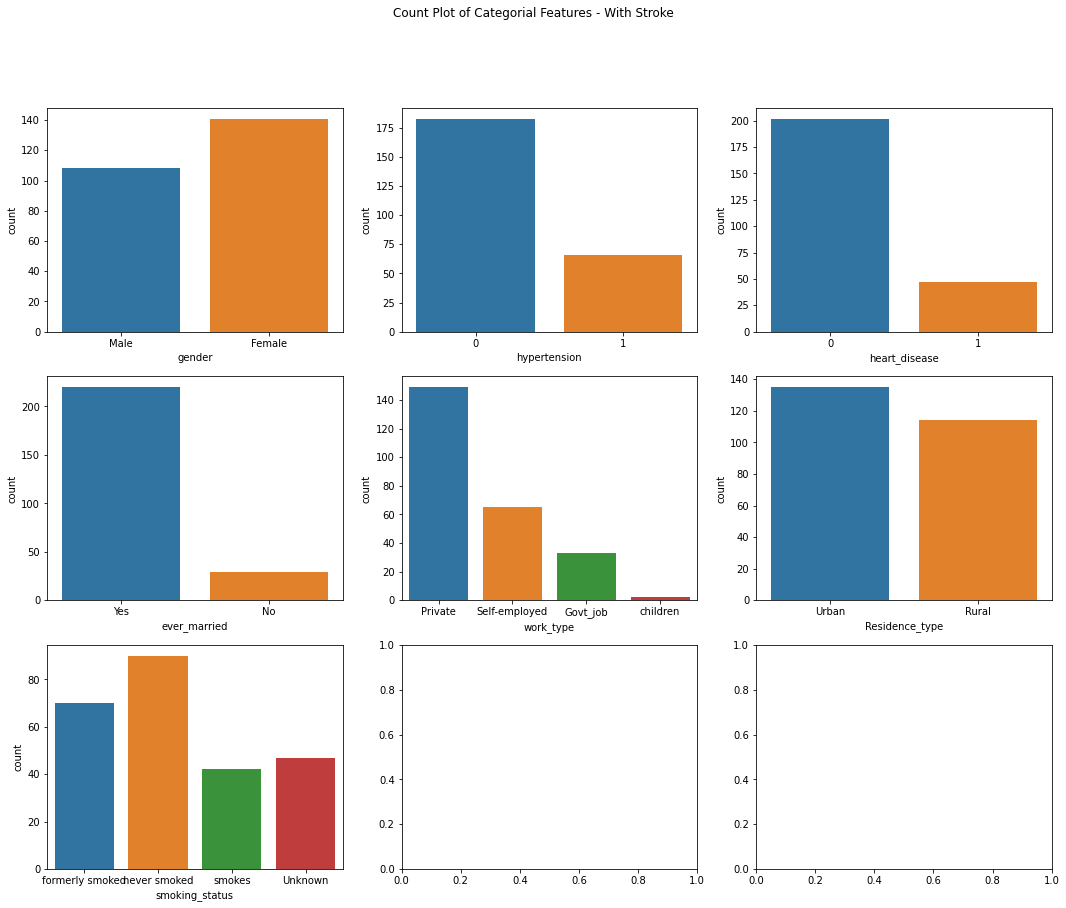Count plot of all categorial features (with stroke)

We can observe that most stroke patients are females with no history of history of hypertension or heart disease. Those who were formerly smokers also have a higher chance of getting a stroke, but those who have never smoked also have a high chance.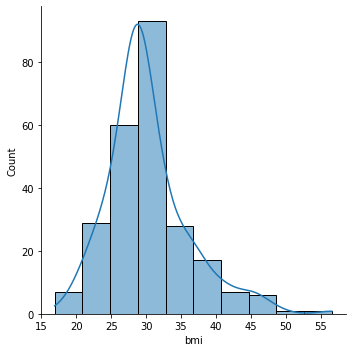Distribution plot of continuous features (with stroke)

In terms of age, the older you are, the higher chances of getting a stroke. Those with a higher glucose level and a BMI between 30–35 are observed to have a higher chance as well.

# Data Preparation

I decided to split the data with a test size of 30% and a random state of 42.

Before building the predictive models, let’s first clean up the data. As we can see in the below figure, the dataset has a disproportionately high percentage of people with no stroke (0 represents patient with no stroke, 1 represents a patient with a stroke)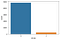Count plot of the target variable (stroke).

To deal with this oversampling, we can use SMOTE. This is what the stroke distribution looks like after balancing the data.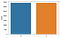Count plot of a stroke after balancing

# Machine Learning Models

The machine learning models I tried were Logistic Regression, Decision Tree, Bagging, Random Forest, and AdaBoost. I also used a Voting Classifier with the above models to compare accuracy scores.

## Logistic Regression

`from sklearn.linear_model import LogisticRegressionfrom sklearn import metricslog_model = LogisticRegression(random_state=42)log_model.fit(X_train, y_train)y_pred_log = log_model.predict(X_test)`

Logistic Regression Accuracy Score: 0.7566862361382909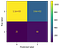Logistic Regression Confusion Matrix

## Decision Tree

`from sklearn.tree import DecisionTreeClassifierdt_model = DecisionTreeClassifier(criterion='entropy',random_state=42)dt_model.fit(X_train, y_train)y_pred_dt = dt_model.predict(X_test)`

Decision Tree Accuracy Score: 0.903457273320287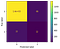Decision Tree Confusion Matrix

## Bagging

`from sklearn.ensemble import BaggingClassifierfrom sklearn.metrics import accuracy_scoremodel_bagging = BaggingClassifier(base_estimator = DecisionTreeClassifier(), n_estimators=10, random_state = 42)model_bagging.fit(X_train, y_train)y_pred_bagging = model_bagging.predict(X_test)`

Bagging Accuracy Score: 0.9347684279191129

Random Forest

`from sklearn.ensemble import RandomForestClassifiermodel_rf = RandomForestClassifier(n_estimators=100, max_features=7, random_state=42)model_rf.fit(X_train, y_train)y_pred_rf = model_rf.predict(X_test)`

Random Forest Accuracy Score: 0.9399869536855838

## Boosting

`from sklearn.ensemble import AdaBoostClassifierbase_est = DecisionTreeClassifier (max_depth =4)ada_boost1 = AdaBoostClassifier(base_est, n_estimators=200, random_state=42, learning_rate=.05)ada_boost1.fit(X_train, y_train)y_pred_ada = ada_boost1.predict(X_test)`

Boosting Accuracy Score: 0.9308545335942596

## Voting Classifier

`logClf = LogisticRegression(random_state=42)dtClf = DecisionTreeClassifier(criterion='entropy',random_state=42)baggingClf = BaggingClassifier(base_estimator = DecisionTreeClassifier(), n_estimators=10, random_state = 42)rfClf = RandomForestClassifier(n_estimators=100, max_features=7, random_state=42)boostClf = AdaBoostClassifier(base_est, n_estimators=200, random_state=42, learning_rate=.05)clf2 = VotingClassifier(estimators = [('log', logClf), ('dt', dtClf), ('bag',baggingClf), ('rf', rfClf), ('boost', boostClf)], voting='soft')clf2.fit(X_train, y_train)clf2_pred = clf2.predict(X_test)`

Voting Classifier Accuracy Score: 0.9275929549902152

The optimal model for this dataset is Random Forest, with an accuracy score of 0.940. Additionally, this model is a good choice because it allows us to identify features that are important and drop any that are irrelevant.

# Fine Tuning Random Forest Model

After finding out the Random Forest Model performs the best, we will then fine tune it with RandomizedSearchCV.

`from sklearn.model_selection import RandomizedSearchCV# fine Tune the model using RandomizedSearchCVparameters= {'n_estimators':[400,500],'max_depth':[7,10],'max_features':[4,5],'min_samples_split' : [100,150],'min_samples_leaf' : [30,40]}rf = RandomForestClassifier()rf_model_tune = RandomizedSearchCV(rf, param_distributions = parameters, cv=3,n_iter = 20, verbose=2, random_state=42)rf_model_tune.fit(X_train,y_train)`

Then using rf_model_tune.best_params_, we get the following best parameters.

Finally, we use these new parameters in the Random Forest model, resulting in a new accuracy score of 0.935.

This new accuracy score is shown to be slightly lower than the original score of 0.940.

# Feature Importance

The top three features affect the stroke probability are as follows:

# Classifying Sample Patient

As a final test on the model, I classified a sample patient with the below characteristics.

Age: 67
Average glucose level: 240
Bmi: 50
Fender: Male
Hypertension: No
Heart Disease: Yes
Ever Married: Yes
Work Type: Private
Residence Type: Urban
Smoking Status: Formerly Smoked

The Random Forest predicted that this sample patient is likely to have a stroke.